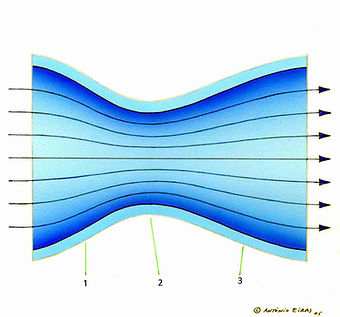## Antonio Eiras

At this point it is essential, for a better understanding of how wings work in developing support forces, a summary explanation of the concepts of the pressures involved.

Thus we have to consider the concept of pressure, in a broad sense, as the applied force per unit area. The static pressure (the first portion of the left side of the Bernoulli equation, presented in the next paragraph) is the force exerted by the particles of the fluid on its surroundings and dynamic pressure (the second portion of the left side of the same equation) results of the fluid displacement. The pressure corresponding to the potential energy (the third and final parcel of the left part of the equation) will not be taken into account since the height variations are not relevant in the context in which we use the equation.

The Bernoulli principle states, so that, in moving fluid, an increase in flow velocity occurs simultaneously with a decrease of the static pressure and/or the potential energy of the fluid. This principle can be written as the equation:

p/ρ + (v^2)/2 + gz = constant

In this equation, p is the pressure at the point chosen for analysis, ρ is the density of the fluid, which remains constant along the flow, v is the fluid velocity tangent at point under consideration, in a streamline, g is the gravity acceleration, and z is the elevation of the same point above a reference plane.

In a simplified form and applied to what interests us to analyze, we can write the equation as follows:

static pressure + dynamic pressure = constant

As we know, by Equation or principle of Bernoulli, when the dynamic pressure increases the static pressure will decrease, and, therefore, we can easily deduce that the static pressure of a fluid is inversely proportional to the square of the speed at which it flows, and that any difference, no matter how small, between the velocities of the air flowing above and below the wing profile will condition a pressure differential between the two surfaces thereof, which results in a lift force perpendicular to the flow direction air and directed, in the case of a inverted wing, down.

If the wing is working near the ground, as we can see in 3b image, the negative lift is going to be, likewise, the resultant force created by the difference of pressures of the air fluxes that flow on both sides of the wing profile.

However, and with the proximity of the ground, there is an accented acceleration of the speed in the airflow that passes under the wing, by the creation of a similar effect like in the Venturi tunnel, between the wing and the track surface.

As we can see in picture 3c, a Venturi tunnel presents a convergent duct (1), with an angle of accented convergence, where the airflow is accelerated, with consequent reduction of the static pressure, which ends in a throat (2), where the speed of the airflow reaches the highest point, and the correspondent static pressure the lowest value, to which it follows a divergent duct (3), with a less accented divergence angle than the convergence one, because the airflow does not tolerate so well the slowing down that it goes through, and where the values of speed and flow pressure are going to, progressive and smoothly, to equal those of the flow as it enters the tunnel, meaning, the air speed is going to slow down and the static pressure of this airflow is going to increase.

This phenomenon, also called “ground effect”, happens, in an obvious way, when the distance wing-ground is inferiors to half of the cord of the wing in study, which could lead to an increase of the negative lift up to 50% more than it would be in “free” airflow, like in the situation described in 3a.

The creation of these differential pressures between the two surfaces of the wings was in the beginning of its use in racing cars, quite limited by a phenomenon that was happening in its side extremities, which was limiting its efficiency. Apparently, and as we can see in the 4a image, due to the air leak of the bigger static pressure zone, in the superior face for the zone of less pressure, it not only annulated one part of this pressures differential, as it also generated two vortexes, created in the wings extremes, which provoked an undesirable ascending or upwash movement of the air that was passing under the wing, and a descending or downwash movement of the air that was passing from it’s both sides (red arrows).